Your research can change the world
More on impact ›

# Frontiers in Astronomyand Space Sciences## Review ARTICLE

Front. Astron. Space Sci., 29 October 2020 | https://doi.org/10.3389/fspas.2020.560149

# Direct and Indirect Measurements for a Better Understanding of the Primordial Nucleosynthesis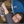Roberta Spartá1,2,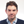Rosario Gianluca Pizzone1*,Carlos A. Bertulani3,Suqing Hou4,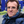Livio Lamia1,2,5 andAurora Tumino1,6
• 1Laboratori Nazionali del Sud, Istituto Nazionale Fisica Nucleare, Catania, Italy
• 2Dipartimento Fisica e Astronomia “Ettore Majorana”, Universitá degli Studi di Catania, Catania, Italy
• 3Department of Physics and Astronomy, Texas A&M University, Commerce, TX, United States
• 4Key Laboratory of High Precision Nuclear Spectroscopy, Institute of Modern Physics, Chinese Academy of Sciences, Lanzhou, China
• 5Centro Siciliano di Fisica Nucleare e Struttura della Materia, Catania, Italy
• 6Facoltá di Ingegneria e Architettura, Universitá degli Studi di Enna “Kore”, Enna, Italy

The Big Bang Nucleosynthesis (BBN) model is a great success of nuclear astrophysics due to the outstanding agreement between observational and predicted light elements abundances. One exception, however, is the so-called “lithium problem.” In this context, experimental efforts to measure the relevant reactions have been brought to an increased level of accuracy in measuring primordial abundances, and the introduction of indirect methods has allowed researchers to overcome the natural limitations of direct measurements in the energy range of interest for BBN. Here we review the results obtained from the application of the Trojan Horse Method to some of the most influential reactions of the standard network, such as 2H(d,p)3H, 2H(d,n)3He, 3He(d,p)4He, 7Li(p,α)4He, and 7Be(n,α)4He. The relevant cross sections have been then used as new inputs to a classical BBN code, resulting in important constraints that make suggestions for a possible solution for the lithium problem outside of nuclear physics.

## 1. Introduction

Big Bang Nucleosynthesis (BBN) occurred when our universe was able to produce nuclei that happened just after the baryogenesis, most probably from the second to the 20th minute after the Bang, while temperature fell from more than 109–108 K. BBN has been widely studied for decades due to its importance for the understanding of the whole Big Bang Model, being one of its three mainstay pieces of evidence, together with the galactic recession and the Cosmic Microwave Background (CMB). As it is the oldest, it is a valuable tool with which to constrain the physical evolution of the Big Bang.

The model actually describing BBN is one of the major successes of nuclear astrophysics as a discipline, especially considering its Standard version (SBBN), which is the most accepted by the community. For this model to be Standard, it is necessary that the baryon-to-photon number densities ratio $\eta =\frac{{n}_{B}}{{n}_{\gamma }}$ is uniform in space and time during BBN; the neutrinos families Nν are three (this is known from the measurement of the Z0 width at CERN Tanabashi et al., 2018), as predicted by the Standard Model for Particle Physics, and no other particle is present in remarkable abundance except of neutrinos ν; And the neutron half-life τn has a value of 879.4 ± 0.6 s (Particle Data Group mean average from 2018 and 2019 updates), which significantly influences the weak interaction rate and the reaction rates of light elements synthesis and destruction. Moreover, SBBN is based on General Relativity and ΛCDM cosmology. Recent and complete reviews for SBBN are given in Cyburt et al. (2016) and Pitrou et al. (2018). This success relies on the outstanding agreement between what is predicted as the output, namely, the primordial abundances of the elements produced during BBN, and the same abundances resulting from the current observations (and brought back with other models to the primordial values). This is true not only for the compliance of nuclear physics and astronomy results but also for the model parameters obtained with methods completely outside of nuclear astrophysics, such as the CMB evaluation from the Planck satellite of η · 10−10 = 6.12 ± 0.06 (Planck Collaboration et al., 2018), impressively concordant with the BBN model result of 5.8 ≤ η · 10−10 ≤ 6.5, which was given in Cooke et al. (2018) and obtained by taking advantage of the most recent and precise measurements of the deuterium primordial abundance. With this recent and precise evaluation of η from the Planck mission, it is now possible to consider SBBN as a parameter-free model, described with computer programs where outputs are the desired primordial abundances and inputs are the cosmological parameters and the rates of the reactions through which light elements are produced.

The only thorn in our side is the Cosmological Lithium Problem, namely the discrepancy of a factor three between what is observed (as an example see Sbordone et al., 2010) and what is predicted by SBBN. Since the early 1980s the Spite plateau, i.e., the nearly constant lithium abundance with decreasing metallicity in halo stars, was assumed as a signature of primordial lithium. Since then, observational developments have confirmed its existence and its predicted cosmologic role. Nevertheless, what is calculated in BBN models is systematically higher than what is observed in halo stars and is assumed to be primordial. In recent times, much effort has been put to shore up the model, and, from an observational point of view, it is now claimed to have reached a precision of a few percent for some elements (Aver et al., 2015; Cooke et al., 2018). Currently, the nuclear measurements part appears to be one of the main sources of uncertainty, as it is difficult to obtain all the involved cross sections with the same degree of precision. Together with nuclear cross sections measurements (some involving the radioactive isotope 7Be interaction with neutron), relevant uncertainties in the prediction of primordial 7Li also arise from stellar physics (e.g., observations, stellar rotation, and transport mechanisms) that are far from being understood.

In fact, to study the origin and evolution of the light element abundances in the galaxy, one should take into account several competing processes besides the Big Bang cosmic-ray production, stellar depletion, and nucleosynthesis all of which are linked to the cosmic and chemical evolution (see e.g., Boesgaard et al., 2004 for a review).

In general, theoretical analyses of light element abundances in stars are still limited by the lack of precise information on the efficiency of envelope convection, microscopic diffusion, and radiative acceleration and on the possible presence of additional mixing mechanisms (e.g., induced by the stellar rotation, see Cayrel et al., 1999). Moreover, the predicted light element depletion strongly depends on the adopted physical input (besides nuclear reaction rates), such as the equation of state and the opacity of the stellar matter, which is still affected by relevant uncertainties (see e.g., Pinsonneault, 1997 for some results of evolutionary models). It is therefore not surprising that discrepancies persist between the predicted and observed light element abundances even for the determination of solar 7Li abundance or in the case of open clusters and halo or disk stars. It is also important to stress that for all the other primordial isotopes, predicted values of abundances and observed ones (in the appropriate astrophysical site) do match.

Despite all the efforts devoted to reduce the uncertainties, in most of the cases, directly measured cross sections are inadequate in the energy range of interest for BBN due to natural limitations, such as the Coulomb barrier presence for charged particle induced reactions, which reduces the cross sections to values so small that they are almost impossible to measure. However, direct measurement data sets have been selected here for all the reactions analyzed with the aim of discerning which set is still valid or not.

Recently, indirect measurements have been performed to overcome these difficulties, particularly using the Trojan Horse Method (THM). This has been applied to some of the most influential reactions of the SBBN network, such as 2H(d,p)3H, 2H(d,n)3He, 3He(d,p)4He, 7Li(p,α)4He as first (Pizzone et al., 2014), and then extended to 7Be(n,α)4He and 3He(n,p)3H. This extension takes advantage of the new applications of the THM to neutron-induced and radioactive-beam-induced reactions, which substantially widens the THM scope to almost all the interesting reactions for astrophysical scenarios. Cross sections thus obtained have been compared with direct measurements and used as new inputs for a classical BBN code. Here we review the main results of the measurements above together with the conclusions that can be drawn from the calculation outcome. In particular, we discuss the resulting constraints that suggest a possible solution for the lithium problem outside of nuclear physics.

## 2. Nuclear Measurement Problems

Particle interaction in the BBN environment took place with thermal energy E ~ kBT as kinetic energy, meaning that 100÷2 keV is the range of astrophysical interest (BBN universe had temperatures T ~ 108÷9 K), which is what laboratory experiments should concentrate on to better understand this first nuclear astrophysics scenario.

Unfortunately, it is very difficult, when not impossible, to have accurate cross section measurements at these energies for charged-particle-induced reactions, as they brutally decrease to values of nano- or pico-barns because of the Coulomb barrier penetration. Measurements are thus very challenging; the background is usually overwhelming, hence the need for extrapolation to the BBN energies. Neutron-induced reactions are instead complicated by the problems related to the production of neutron beams with sufficient intensity and energy precision to be helpful for astrophysical aims.

Extrapolation of the S(E) (the astrophysical factor) rather than the cross section is one of the means used to reach these very low energies because it keeps a nearly constant trend in cases of a non-resonant reaction. But the extrapolation cannot be a complete solution, as it does not solve cases with resonances and is not helpful in evaluating electron screening enhancement at ultra-low energies. Moreover, extrapolation can be source of a huge error because the presence and the effects of under-threshold resonances can be unknown.

Indirect measurements are complementary to the direct ones and have proven to always be more helpful in completing our knowledge on the astrophysically interesting yields. Among them, the Trojan Horse Method (THM) provides bare nucleus cross section without electron screening but mostly without suppression effects due to the Coulomb barrier (or centrifugal barrier in case of n-induced reactions), as discussed in detail in the review by Spitaleri et al. (2016, 2019).

The THM allows us to cover a wide energy range using only one beam energy (see for instance, Sergi et al., 2015; Cvetinović et al., 2018; Rapisarda et al., 2018). For this reason, the THM is used not only in contexts where light elements are present, as in the case of BBN scenario, but also for studying heavier species interaction, like 12C + 12C (Tumino et al., 2018), which are fundamental for stellar physics. Moreover, in recent years, it has been applied to measure n-induced reactions, bypassing all the problems related to the neutron beam production using deuterons as source of virtual neutrons (Lamia et al., 2008; Gulino et al., 2010; Guardo et al., 2017). On top of that, THM applications to radioactive ion beams (Cherubini et al., 2015; Pizzone et al., 2016) have paved the way to the unique possibility of studying the interaction between exotic beams and neutrons. We have also mentioned a recent measurement of the 3He(α,γ)7Be, by means of the ANC method, which has provided a new value of S(E = 0 = 0.534 ± 0.025 keV · b (see reference Kiss et al., 2020 for details).

## 3. THM Measurements for the BBN Scenario

Some of the most influential reactions for SBBN (as an example see Cyburt et al., 2016), i.e., 2H(d,p)3H, 2H(d,n)3He, 3He(d,p)4He, 7Li(p,α)4He, 7Be(n,α)4He, have been investigated using the THM in the energy range of interest, and their measurements were performed in an experimental campaign that took place within the last decade (Pizzone et al., 2003; La Cognata et al., 2005; Tumino et al., 2011). We will not go into the details of the THM because this is done elsewhere (see Spitaleri et al., 2003; Spitaleri et al., 2016, 2019; Tumino et al., 2013 and references therein), but it is necessary to recall that the THM provides a bare S(E), i.e., an astrophysical factor that is lacking in screening and barrier effects (Coulomb or centrifugal), for the reaction under investigation after studying an appropriate three-body one in the quasi-free (QF) kinematical conditions. The basic idea of the THM is to get the cross section at low energies of a two-body reaction, which is interesting for astrophysical scenarios:

extracting it by means of the QF mechanism of a proper three-body reaction

Consequently, we will measure the cross section of the a nucleus interacting with A, which is composed by the two clusters x, participating to the binary reaction in Equation (2), and s, namely the residual nucleus, or spectator, which will not take part in the binary reaction. The break-up of A is QF when s is emitted with the same momentum it had inside A.

Once the QF break up mechanism is disentangled from all other reaction mechanisms, and through the use of Plane Wave Impulse Approximation (PWIA), the three-body cross section can be factorized:

where the kinematical factor KF generally comes from Monte Carlo simulation, which considers the detectors geometrical position, while $|{\varphi }_{exp}\left({p}_{s}\right){|}^{2}$ is the momentum distribution of the spectator particle. From Equation (3) one can extract the HOES cross sections, ${\frac{d\sigma }{d\Omega }}_{Cc}^{HOES}$, and then normalize it to OES one above the Coulomb barrier to the directly measured data. This cross section for the binary reaction a + xc + C can be obtained as a function of the relative energy Eax, given by Eax = ECcQ2b (where Q2b is the Q value of the binary reaction) from energy conservation. Indeed, in QF kinematics when px = 0, it results in the following:

This explains how the two-body reaction can be induced at such low energies, exploiting the compensation of the interaction energy for the TH nucleus binding energy.

Considering the temperatures of the universe at SBBN time, measurements of cross sections are interesting at energies of 101÷2 keV. The possibility to investigate this energy range with a unique beam energy is allowed by measuring small deviations from QF conditions. This means that Equation (4) becomes the following:

A small variation of the ps value and/or of the θs (the angle where the spectator is emitted) thus makes it possible to scan most of the desired energy range.

For the examined reactions, the S(E) factors were normalized and then compared with those available from direct measurements in literature. They have showed to be in fair agreement in the energy region where screening effects are negligible.

### 3.1. R-Matrix Fit

Details of this fit procedure are given in Pizzone et al. (2014).

Here we just recall that these fits have been done by means of AZURE, the multilevel and multi-channel R-matrix public code (Azuma et al., 2010). We used direct data together with THM data in the energy range where they were available and used only direct data where not, to fit the R-matrix output of the reactions in section 3. The parameterization of these functions has considered nR resonances, where Ej [MeV] are the resonance energies and Γj [MeV] the widths, which are the sum of polynomials and Breit-Wigner functions:

in [MeV · b]. We considered the ordinary χ2 statistics, as explained in Pizzone et al. (2014).

### 3.2. 2H(d,p)3H

The d + d reactions are among the most influential processes on the final abundance output, being at the base of the reaction chain that leads to the light element production. For this reason and for their interest in energy production with fusion, many measurements are available in the literature for each of the two mirror channels, 2H(d,p)3H and 2H(d,n)3He. We updated for each reaction the data sets from direct measurement to be compared with the THM one, to better estimate the impact of the indirect result. For the pt channel we have chosen data reported in Greife et al. (1995), Krauss et al. (1987), McNeill and Keyser (1951), Schulte et al. (1972), Brown and Jarmie (1990), Ganeev et al. (1957), Arnold et al. (1954), Raiola et al. (2002), Booth et al. (1956), Davenport et al. (1953), Von Engel and Goodyear (1961), Cook and Smith (1953), Moffatt et al. (1952), Tie-Shan et al. (2007), and Leonard et al. (2006).

The data set in Greife et al. (1995) shows an enhanced S-factor at very low energy values due to the electron screening effect. This effect has to be removed in order for us to use data sets for astrophysical applications. It is also noticeable that any data set is present at energies of 1 MeV, so the fitting procedure can hardly be reliable.

Experimental runs to extract the TH bare nucleus S-factor from three-body reaction 2H(3He,pt)H, with their data analysis started with an early work of Rinollo et al. (2005) and were followed by Tumino et al. (2011), Pizzone et al. (2013), and Tumino et al. (2014). In these latter works the energy range covered span from 2.6 keV up to 1.5 MeV with a 5% error. The TH result is shown in Figure 1 (blue filled circles) together with the data sets by direct measurements (red circles), used for comparison. The R-matrix fit to both direct and indirect data is indicated by a solid line, with parameters for an equivalent polynomial fit, using Equation (6), listed in Table 1.

FIGURE 1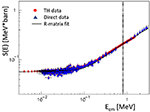Figure 1. Bare nucleus S-factor for the 2H(d,p)3H reaction obtained after putting together direct data (blue filled triangles) and with the THM ones (red filled circles) taken from (Tumino et al., 2014). The solid line is the R-matrix fit to direct and indirect sets, as in section 3.1. The resulting parameters of the equivalent fit (polynomial plus Breit-Wigner) are listed in Table 1. Energy range of interest for BBN is marked by the vertical dotted line. The figure is adapted from Pizzone et al. (2014).

TABLE 1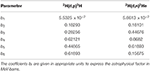Table 1. Fit parameters for the S-factors of the reactions 2H(d,p)3H and 2H(d,n)3He measured in TH experiments using Equation (6).

### 3.3. 2H(d,n)3He

The n3He is the mirror channel of the previous reaction, and it is therefore not surprising that available literature measurements depict a state of the art before the THM measurement very similar to it, including that data sets are missing between 600 keV and 1 MeV. But, unlike the pt case, no experimental points in absolute units are present below 6 keV. We thus used data sets from Greife et al. (1995), Krauss et al. (1987), McNeill and Keyser (1951), Schulte et al. (1972), Brown and Jarmie (1990), Ganeev et al. (1957), Arnold et al. (1954), Raiola et al. (2002), Booth et al. (1956), Leonard et al. (2006), Davidenko et al. (1957), Hofstee et al. (2001), Preston et al. (1954), Belov et al. (1990), Ying et al. (1973), and Bystritsky et al. (2010).

TH bare nucleus S-factor obtained extracting the quasi-free mechanism from the 2H(3He,n3He)H (Tumino et al., 2011, 2014) is shown in Figure 2, with a 5% experimental error on the whole data set, from 2.6 keV up to 1.5 MeV, as blue filled triangles and red filled triangles are direct measurements. Also in this case the solid lines are the R-matrix fits (to direct and indirect data), with parameters for an equivalent polynomial fit, using Equation (6), listed in Table 1.

FIGURE 2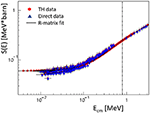Figure 2. Bare nucleus S-factor for the 2H(d,n)3He reaction obtained after putting together direct data (blue filled triangles) and with the THM ones (red filled circles) taken from Tumino et al. (2014). The solid line is the R-matrix fit to direct and indirect sets, as in section 3.1. The resulting parameters of the equivalent fit (polynomial plus Breit-Wigner) are listed in Table 1. Energy range of interest for BBN is marked by the vertical dotted line. The figure is adapted from Pizzone et al. (2014).

### 3.4. 3He(d,p)4He

The 3He(d,p)4He fusion reaction is important in the ultra-low energy range for many different topic, e.g., Solar Physics, cosmology, pure, and applied physics. Its cross section was measured in the Gamow energy region by several authors through direct methods. For the 3He(d,p)4He we used the direct data from Engstler et al. (1988), Krauss et al. (1987), Bonner et al. (1952), Zhichang et al. (1977), Geist et al. (1999), Möller and Besenbacher (1980), Erramli et al. (2005), Schroeder et al. (1989), and Aliotta et al. (2001). Together with other indirect methods, the THM also offered an alternative approach by means of a dedicated experiment which was performed using 6Li as a Trojan Horse nucleus and extracting data from the quasi-free break-up channel to the 3He(6Li,pα)4He reaction. The astrophysical factor was consequently measured for Ecm = 0 ÷ 1 MeV and fitted following Equation (6), as reported in La Cognata et al. (2005). The result is portrayed in Figure 3 with red solid dots for THM data and full blue triangles for the direct data (Bonner et al., 1952; Krauss et al., 1987; Geist et al., 1999; Aliotta et al., 2001). The solid line is an R-matrix fit to the direct and THM data, as described in section 3.1. The coefficients for the polynomial plus Breit-Wigner fit are reported in Table 2.

FIGURE 3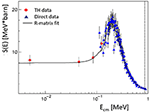Figure 3. Bare nucleus S(E)-factor for the 3He(d,p)4He process obtained with direct data (blue filled triangles) and with the THM (red filled dots) taken from La Cognata et al. (2005). Full description is reported in the text. The figure is adapted from Pizzone et al. (2014).

TABLE 2Table 2. Table of fit parameters for the S-factors of the reactions 3He(d,p)4He and 7Li(p,α)4He measured in TH experiments using Equation (6).

### 3.5. 7Li(p,α)4He

The process that contributes most to Li destruction in cosmic environments is the 7Li(p,α)4He. This is therefore the determinant in the challenging scenarios of both primordial and stellar lithium destruction. In the BBN the discrepancy of about a factor of three between its predictions and the observed Li abundances in halo stars represents the well-known and still debated “cosmological lithium problem.” Many possible reasons for this discrepancy were suggested, from stellar depletion to non-standard Big Bang Nucleosynthesis models.

The 7Li(p,α)4He process was studied accurately in the last three decades either by means of direct methods (Engstler et al., 1988; Cruz et al., 2009) as well as by indirect ones (Aliotta et al., 2000; Lattuada et al., 2001; Pizzone et al., 2003; Lamia et al., 2012b), using the THM.

For this reaction we used data arising from the measurements performed by Schroeder et al. (1989), Mani et al. (1964), Cassagnou et al. (1962), Fiedler and Kunze (1967), Spinka et al. (1971), Rolfs and Kavanagh (1986), and Harmon (1989) as well as Engstler et al. (1992), Ciric et al. (1976), Spraker et al. (1999), Lee (1969), and Cruz et al. (2009). The most recent data set for the S(E) factor for this reaction, obtained with the THM after d quasi-free breakup, are plotted in Figure 4 (Lamia et al., 2012a) as red dots while the direct ones are reported as blue triangles. The solid line represents a R-matrix fit to both direct and indirect data following the prescription in section 3.1. The parameters for an equivalent polynomial expansion are reported in Table 2.

FIGURE 4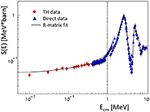Figure 4. Bare nucleus S(E)-factor for the 7Li(p,α)4He reaction obtained with direct data (blue triangles) and with the THM (red dots) taken from Lamia et al. (2012b). The solid line is an R-matrix fit to the overall direct and indirect data sets as discussed in the text. The figure is adapted from Pizzone et al. (2014).

### 3.6. 7Be(n,α)4He

The evaluation of this reaction rate has been the subject of many efforts, being one of the most influential on the 7Li primordial abundance; it is simultaneously a difficult measurement, as it involves a neutron and a radioactive nucleus. For this reason literature data sets available are very few, and indirect methods have proved to be very helpful in this case (Hou et al., 2015; Kawabata et al., 2017). The use of the THM has led to a first reaction rate evaluation (Lamia et al., 2017) in which the cross section was derived from two measurements of 7Li(p,α)4He (described in section 3.5) using the charge symmetry hypothesis. Later, the S(E) of the 7Be(n,α)4He was obtained using the THM applied to the 2H(7Be,α 4He)H measurement, performed at the EXOTIC facility, whose results are shown in Lamia et al. (2019). Here the experimental difficulties are partially overcome by the use of the deuteron target as a neutron virtual inducer, as already successfully tested in many other TH experiments (Lamia et al., 2008; Gulino et al., 2010; Spartá, 2016; Guardo et al., 2017).

The obtained reaction rate is shown in Figure 5 as a ratio to the one obtained with the direct measurement of Hou et al. (2015), where they appear in fair agreement but the uncertainty is reduced in the indirect measurement.

FIGURE 5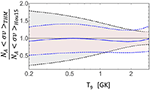Figure 5. Ratio of indirect (Lamia et al., 2019) on the direct (Hou et al., 2015) rates for 7Be(n,α)4He reaction, as a function of T9. Rates are in agreement and the uncertainty is reduced thanks to the indirect measurement. The figure is adapted from Lamia et al. (2019).

### 3.7. 3He(n,p)3H

The 3He(n,p)3H reaction is one of the most important neutron-induced processes in BBN and has an important impact on the primordial 3He and 7Li abundances. At the temperatures important for predicting Big Bang yields, the reaction rate is determined by the cross section in the energy range 0.03 ≤ Ecm ≤0.3 MeV. The first studies of this reaction were performed by Coon (1950) in the 0.1 ≤ Ecm ≤ 30 MeV using a neutron beam. Errors turned out to be around 30%. Other measurements, more focused at lower energies, were conducted by Batchelor et al. (1955) (direct one, 0.1 ≤ Ecm ≤ 1 MeV), Gibbons and Macklin (1959) (inverse measurement with larger uncertainties), and Costello et al. (1970) who measured directly in the range 0.3 ≤ Ecm ≤ 1.1 MeV. The most recent data belong to Drosg and Otuka (2015) in a wide energy range. Reaction rates were then calculated for astrophysical applications by Brune et al. (1999) and Adahchour and Descouvemont (2003), which show a similar trend at temperatures of astrophysical interest while the reaction rate calculated by Caughlan and Fowler (1988) is sensitively higher. In the energy range of interest, the existing data are therefore sparse and mostly measured more than 50 years ago after facing tough experimental challenges, several times resulting in errors as high as 30%. This is clearly reported in Figure 6.

FIGURE 6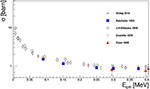Figure 6. Present day measurements of the 3He(n,p)3H cross section performed by different methods as reported in the text.

A measurement was recently performed by means of the THM with the same methodology reported for the other reactions. Data analysis is still in progress (Pizzone et al., 2020; Spampinato et al., 2020). A preliminary reaction rate, calculated upon the data shown in Pizzone et al. (2020) is plotted in Figure 7 with a comparison with the reaction rate published by Brune et al. (1999). In the energy region important for astrophysics a deviation up to 20% is present and will be investigated in future works.

FIGURE 7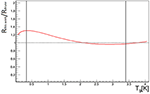Figure 7. Reaction rate for the 3He(n,p)3H calculated from THM data (red line) reported in Pizzone et al. (2020) compared with the calculations from Brune et al. (1999). The black vertical lines mark the temperature interval important for BBN.

### 3.8. From TH Data to Reaction Rates

These new compilation of direct and THM data has led to the numerical calculation of new reaction rates for the reactions in sections above, introducing the R-matrix fits in the reaction rate definition.

Then, these rates have been fitted with the Equation (7),

as it is the common procedure adopted in previous works (as for example Smith et al., 1993; Cyburt, 2004; Coc et al., 2012).

Equation (7) contains the relevant temperature dependence of the reaction rates during the BBN. Moreover, we also got the respective uncertainties in the rates including experimental errors in the calculations.

In Table 3 the ai coefficients for the 2H(d,p)3H and 2H(d,n)3He are listed, while the same are listed for 3He(d,p)4He and 7Li(p,α)4He in Table 4. The odd columns consider the rates coming from both THM and direct measurements, while the even ones consider the rates coming from the direct data sets (see next section for details).

TABLE 3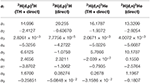Table 3. Parameters of the reaction rates of Equation (7) for 2H(d,p)3H and 2H(d,n)3He evaluated from the S-factors from TH + direct measurements and from direct measurements.

TABLE 4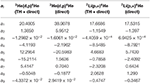Table 4. Parameters of the reaction rates of Equation (7) for 2H(d,p)3H and 2H(d,n)3He evaluated from the S-factors from TH + direct measurements and from direct measurements.

To avoid the enhancement due to the electron screening, it is worth noticing that direct data for 3He(d,p)4He and 7Li(p,α)4He were considered for energies above 100 keV and above 10 keV for 2H(d,p)3H and 2H(d,n)3He.

Figures 8, 9 show the ratio of the THM reaction rates (“TH”) with those from other compilations, meaning our own fit to existing direct reaction capture data (“Direct”), the NACRE compilation (Angulo et al., 1999) (“Nacre”), from Smith et al. (1993) (SKM), and from Cyburt (2004) (“Cyb04”). Here the error bands are related to the error bars of the associated THM + direct S-factors.

FIGURE 8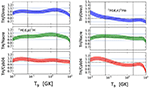Figure 8. (Left) Trojan Horse 2H(d,p)3H reaction rates as a ratio to the one obtained from direct data fit in the upper panel; the middle and lower panels show rates as a ratio to rates published in NACRE (Angulo et al., 1999; Cyburt, 2004). Lower and upper temperature of interest for BBN are marked by the vertical lines. Blue lines define the ±10% deviation from the unity. The figure is adapted from Pizzone et al. (2014). (Right) Same reaction rates ratios as in the left figure but for 2H(d,n)3He. The figure is adapted from Pizzone et al. (2014).

FIGURE 9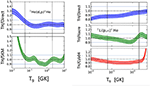Figure 9. (Left) Trojan Horse 3He(d,p)4He reaction rates as a ratio to the one obtained from direct data fit in the upper panel; the middle panel shows the same as a ratio to rate in Smith et al. (1993). Lower and upper temperature of interest for BBN are marked by the vertical lines. Blue lines define the ±10% deviation from the unity. The figure is adapted from Pizzone et al. (2014). (Right) 7Li(p,α)4He reaction rates ratios from THM to the one obtained from direct data (upper panel), while in the middle and lower panels there TH rates ratios with rates published in NACRE (Angulo et al., 1999; Cyburt, 2004). Lower and upper temperatures of interest for BBN are marked by the vertical lines. Blue lines define the ±10% deviation from the unity. The figure is adapted from Pizzone et al. (2014).

For the four reactions considered, deviations up to 20% from previous compilations have been obtained, and for 7Li(p,α)4H above T9 ~ 4, a very large discrepancy with the reaction rate by Cyburt (2004) was found.

The 7Be(n,α)4He rate, discussed in section 3.6, has been compared to the direct and recent one in Hou et al. (2015), with the ratio of the two rates is shown in Figure 5. The TH rate is reported in tabular form in Table 5 as a function of T9 and with the Lower and Upper values, which take into account the error bars.

TABLE 5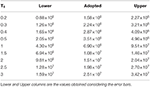Table 5. Reaction rate table for 7Be(n,α)4He calculated from the TH measurement in Lamia et al. (2019) (Adopted) as a function of T9 and expressed in $\frac{c{m}^{3}}{mol·s}$.

## 4. Results

The cross section measurements obtained with THM in the energy range of interest for BBN were used to evaluate the relative reaction rates; the parameters are listed in Tables 35. These have been put in a revised BBN code originally from Kawano and discussed in Pizzone et al. (2014).

The observational 4He mass fraction, Yp = 0.2565 ± 0.006 is from Izotov and Thuan (2010). The deuterium abundance is the mean average equivalent to Ω · ${\text{h}}_{BBN}^{2}$ = 0.0213 ± 0.0013 from O'Meara et al. (2006). As for the 3He, the abundance is adopted from Bania et al. (2002) as a lower limit to the primordial abundance; finally, 7Li abundance is reported in Sbordone et al. (2010) from the observations of lithium plateau stars.

Primordial abundances are then calculated using different reaction rate sets: the first column of Table 6 shows the results from our own fit to the world direct data, while in the second column direct data for 2H(d,p)3H has been replaced by TH data from Tumino et al. (2014) (in section 3.2).

TABLE 6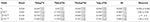Table 6. Primordial abundances as predicted by the Kawano code changing the reaction rate sets (described in the text) and compared with the observational results (last column).

The same holds for the other columns, adopting the reaction rate for 2H(d,n)3He (section 3.3), 3He(d,p)4He (section 3.4), and 7Li(p,α)4He (section 3.5), respectively, keeping the rest of the rates coming from direct measurements. The values in the column marked with All are obtained using the four TH reaction rates described in the sections mentioned above. These values from the code are affected by an uncertainty due to the propagation of the experimental errors. Finally, they can be compared to the last column, which reports the primordial abundances from observations.

Similar calculations have been carried out also for the 7Be(n,α)4He reaction in order to get the primordial values of lithium and beryllium abundances. Table 7 reports results from different calculations. In particular, 7Be(n,α)4 rates from different sources have been included in the THM rates reported in Pizzone et al. (2014): from Hou et al. (2015) in the first line, from Lamia et al. (2017) the second line, and from Lamia et al. (2019) in the third line.

TABLE 7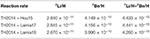Table 7. Predicted primordial abundances of 7Li, 7Be, and their sum, inserting reaction rates from Pizzone et al. (2014) (Table 6) and the 7Be(n,α)4He from Hou et al. (2015) in the first line, Lamia et al. (2017) in the second, and Lamia et al. (2019) in the third.

## 5. Conclusions

Primordial abundances in Table 6 are obtained from direct and indirect reaction rates, while values in the last column refer to the observations. It can be seen that no relevant changes are obtained with these different sets of rates.

A similar result is the one proposed in Table 7, where it is worth noticing that all the three values calculated for lithium abundances are still larger than the one resulting from halo-stars observation in Sbordone et al. (2010) of ${1.58}_{-0.28}^{+0.35}$. This definitely leads to the idea that the cosmological lithium problem is not imputable to systematic errors in nuclear measurements, and no nuclear solution to the cosmological lithium problem can be foreseen. Proposed alternative ideas can be found in Bertulani (2019) or Mathews et al. (2020). Further measurements, such as the 7Be(n,p)7Li with THM, will probably be helpful to strengthen this result.

## Author Contributions

Nuclear data were collected, elaborated, and fitted by RS and RP. LL and SH took care of the 7Be(n,p)7Li data analysis and the relative reaction rate calculation. RP, AT, RS, and LL analyzed the THM data available in the present paper. CB and SH performed the primordial nucleosynthesis calculations. The paper was written by RS and corrected by all authors.

## Funding

SH was supported by the National Natural Science Foundation of China under Grant No. 11705244 and the Youth Innovation Promotion Association of Chinese Academy of Sciences under Grant No. 2019406. CB was supported by U.S. DOE Grant No. DE-FG02-08ER41533 and the U.S. NSF Grant No. 1415656. This work has been partially supported by grant Finanziamenti di linea 2 and Starting grant 2020 by University of Catania.

## Conflict of Interest

The authors declare that the research was conducted in the absence of any commercial or financial relationships that could be construed as a potential conflict of interest.

## References

Adahchour, A., and Descouvemont, P. (2003). R-matrix analysis of the 3He(n, p)3H and 7Be(n, p)7Li reactions. J. Phys. G Nucl. Phys. 29, 395–403. doi: 10.1088/0954-3899/29/2/315

Aliotta, M., Raiola, F., Gyürky, G., Formicola, A., Bonetti, R., Broggini, C., et al. (2001). Electron screening effect in the reactions 3He(d,p)4He and d(3He,p)4He*. Nucl. Phys. A 690, 790–800. doi: 10.1016/S0375-9474(01)00366-9

Aliotta, M., Spitaleri, C., Lattuada, M., Musumarra, A., Pizzone, R. G., Tumino, A., et al. (2000). Improved information on electron screening in 7Li(p,α)α using the Trojan-horse method. Eur. Phys. J. A 9, 435–437. doi: 10.1007/s100500070001

Angulo, C., Arnould, M., Rayet, M., Descouvemont, P., Baye, D., Leclercq-Willain, C., et al. (1999). A compilation of charged-particle induced thermonuclear reaction rates. Nucl. Phys. A 656, 3–183. doi: 10.1016/S0375-9474(99)00030-5

Arnold, W. R., Phillips, J. A., Sawyer, G. A., Stovall, E. J., and Tuck, J. L. (1954). Cross sections for the reactions D(d, p)T, D(d, n)He3, T(d, n)He4, and He3(d, p)He4 below 120 keV. Phys. Rev. 93, 483–497. doi: 10.1103/PhysRev.93.483

Aver, E., Olive, K. A., and Skillman, E. D. (2015). The effects of He I λ10830 on helium abundance determinations. J. Cosmol. Astropart. Phys. 2015:011. doi: 10.1088/1475-7516/2015/07/011

Azuma, R. E., Uberseder, E., Simpson, E. C., Brune, C. R., Costantini, H., de Boer, R. J., et al. (2010). AZURE: an R-matrix code for nuclear astrophysics. Phys. Rev. C 81:045805. doi: 10.1103/PhysRevC.81.045805

Bania, T., Rood, R. T., and Balser, D. S. (2002). The cosmological density of baryons from observations of 3 He+ in the milky way. Nature 415, 54–57. doi: 10.1038/415054a

Batchelor, R., Aves, R., and Skyrme, T. H. R. (1955). Helium-3 filled proportional counter for neutron spectroscopy. Rev. Sci. Instr. 26, 1037–1047. doi: 10.1063/1.1715182

Belov, A. S., Kusik, V. E., and Ryabov, Y. V. (1990). The nuclear fusion for the reactions 2H(d,n)3He, 2H(d,γ)4He at low deuterons energy and cold nuclear fusion. Il Nuovo Cim. A 103, 1647–1650. doi: 10.1007/BF02820309

Bertulani, C. A. (2019). Big bang nucleosynthesis and the lithium problem. J. Phys. Conf. Series 1291:012002. doi: 10.1088/1742-6596/1291/1/012002

Boesgaard, A. M., McGrath, E. J., Lambert, D. L., and Cunha, K. (2004). Boron benchmarks for the galactic disk. Astrophys. J. 606, 306–318. doi: 10.1086/382672

Bonner, T. W., Conner, J. P., and Lillie, A. B. (1952). Cross section and angular distribution of the He3(d,p)He4 nuclear reaction. Phys. Rev. 88:473. doi: 10.1103/PhysRev.88.473

Booth, D. L., Preston, G., and Shaw, P. F. D. (1956). The cross section and angular distributions of the dd reactions between 40 and 90 keV. Proc. Phys. Soc. A 69:265. doi: 10.1088/0370-1298/69/3/309

Brown, R. E., and Jarmie, N. (1990). Differential cross sections at low energies for H2(d,p)3D and h2(d,n)3He. Phys. Rev. C 41:1391. doi: 10.1103/PhysRevC.41.1391

Brune, C. R., Hahn, K. I., Kavanagh, R. W., and Wrean, P. R. (1999). Total cross section of the 3 H (p, n) 3 he reaction from threshold to 4.5 MeV. Phys. Rev. C 60:015801. doi: 10.1103/PhysRevC.60.015801

Bystritsky, V. M., Gerasimov, V. V., Krylov, A. R., Parzhitsktii, S. S., Il'guzin, D. A., Ananin, P. S., et al. (2010). Using a hall accelerator to investigate d(d,n)3He and d(p,γ)3He reactions in the astrophysical energy region. Bull. Russ. Acad. Sci. Phys. 74, 531–534. doi: 10.3103/S1062873810040234

Cassagnou, Y., Jeronymo, J. M. F., Mani, G. S., Sadeghi, A., and Forsyth, P. D. (1962). The li7(p,α)α reaction. Nucl. Phys. 33, 449–457. doi: 10.1016/0029-5582(62)90537-0

Caughlan, G. R., and Fowler, W. A. (1988). Thermonuclear reaction rates v. Atomic Data Nucl. Data Tables 40, 283–334. doi: 10.1016/0092-640X(88)90009-5

Cayrel, R., Spite, M., Spite, F., Vangioni-Flam, E., Cassé, M., and Audouze, J. (1999). New high S/N observations of the (6Li)/(7) Li blend in HD 84937 and two other metal-poor stars. arXiv astro-ph/9901205.

Cherubini, S., Gulino, M., Spitaleri, C., Rapisarda, G. G., La Cognata, M., Lamia, L., et al. (2015). First application of the trojan horse method with a radioactive ion beam: Study of the 18f(p,α)15o reaction at astrophysical energies. Phys. Rev. C 92:015805. doi: 10.1103/PhysRevC.92.015805

Ciric, D. M., Popic, R. V., Zakula, R. B., Stepancic, B. Z., Aleksic, M. R., and Seytajcic, J. P. (1976). The interaction of 7Li isotope with low energy proton and triton beams. Rev. Sci. Res. Czech Rep. 6:115.

Coc, A., Goriely, S., Xu, Y., Saimpert, M., and Vangioni, E. (2012). Standard big bang nucleosynthesis up to CNO with an improved extended nuclear network. Astrophys. J. 744:158. doi: 10.1088/0004-637X/744/2/158

Cook, C. F., and Smith, J. R. (1953). Cross section for the reaction D(d,p)H3. Phys. Rev. 89:785. doi: 10.1103/PhysRev.89.785

Cooke, R. J., Pettini, M., and Steidel, C. C. (2018). One percent determination of the primordial deuterium abundance. Astrophys. J. 855:102. doi: 10.3847/1538-4357/aaab53

Coon, J. H. (1950). Disintegration of he3 by fast neutrons. Phys. Rev. 80:488. doi: 10.1103/PhysRev.80.488

Costello, D. G., Friesenhahn, S. J., and Lopez, W. M. (1970). 3He(n,p)t cross section from 0.3 to 1.16 MeV. Nucl. Sci. Eng. 39, 409–410. doi: 10.13182/NSE70-A20005

Cruz, J., Fonseca, M., Luis, H., Mateus, R., Marques, H., Jesus, A., et al. (2009). Production and characterization of thin 7Li targets fabricated by ion implantation. Nucl. Instr. Methods Phys. Res. B 267, 478–481. doi: 10.1016/j.nimb.2008.11.028

Cvetinović, A., Spitaleri, C., Spartá, R., Rapisarda, G. G., Puglia, S. M. R., La Cognata, M., et al. (2018). Trojan horse measurement of the b 10 (p, α 0) be 7 cross section in the energy range from 3 keV to 2.2 MeV. Phys. Rev. C 97:065801. doi: 10.1103/PhysRevC.97.065801

Cyburt, R. H. (2004). Primordial nucleosynthesis for the new cosmology: determining uncertainties and examining concordance. Phys. Rev. D 70:023505. doi: 10.1103/PhysRevD.70.023505

Cyburt, R. H., Fields, B. D., Olive, K. A., and Yeh, T.-H. (2016). Big bang nucleosynthesis: present status. Rev. Mod. Phys. 88:015004. doi: 10.1103/RevModPhys.88.015004

Davenport, P. A., Jeffries, T. O., Owen, M. E., Price, F. V., and Roaf, D. (1953). The dd cross-section and angular distribution between 55 and 430 keV. Proc. R. Soc. Lond. A Math. Phys. Sci. 216, 66–71. doi: 10.1098/rspa.1953.0007

Davidenko, V. A., Kucher, A. M., Pogrebov, I. S., and u, F. T. J. (1957). Total cross section measurement of D(d,n)3He reaction in energy range 20 to 220 keV. Soviet Atomic Energy Suppl. 5:7.

Drosg, M., and Otuka, N. (2015). Evaluation of the Absolute Angle-Dependent Differential Neutron Production Cross Sections by the Reactions 3H(p,n)3He, 1H(t, n)3He, 2H(d,n)3He, 3H(d,n)4He, and 2H(t,n)4He and of the Cross Sections of Their Time-Reversed Counterparts Up to 30 MeV and Beyond. INDC (AUS)-0019 (Vienna: International Atomic Energy Agency).

Engstler, S., Krauss, A., Neldner, K., Rolfs, C., Schröder, U., and Langanke, K. (1988). Effects of electron screening on the 3He(d,p)4He low-energy cross sections. Phys. Lett. B 202, 179–184. doi: 10.1016/0370-2693(88)90003-2

Engstler, S., Raimann, G., Angulo, C., Greife, U., Rolfs, C., Schröder, U., et al. (1992). Test for isotopic dependence of electron screening in fusion reactions. Phys. Lett. B 279, 20–24. doi: 10.1016/0370-2693(92)91833-U

Erramli, H., Sauvage, T., Rhazi, M., and Misdaq, M. A. (2005). Measurement of the 3He(d,p)4He nuclear reaction cross section by coincidence detection of alphas and protons: application to the determination of the 3He desorption rate from helium bubbles in silicon. Phys. Chem. News 23, 67–72.

Fiedler, O., and Kunze, P. (1967). Wirkungsquerschnitte der kernreaktionen 6Li(p,α)3He und 7Li(p,α)4He bei kleinsten energien. Nucl. Phys. A 96, 513–520. doi: 10.1016/0375-9474(67)90601-X

Ganeev, A. S., Govorov, A. M., Osetinskii, G. M., Rakivnenko, A. N., Sizov, I. V., and S, S. V. (1957). The D-D reaction in the deuteron energy range 100–1000 keV. Soviet Atomic Energy Suppl. 5:21.

Geist, W. H., Brune, C. R., Karwowski, H. J., Ludwig, E. J., Veal, K. D., and Hale, G. M. (1999). The 3He(d,p)4He reaction at low energies. Phys. Rev. C 60:054003. doi: 10.1103/PhysRevC.60.054003

Gibbons, J. H., and Macklin, R. L. (1959). Total neutron yields from light elements under proton and alpha bombardment. Phys. Rev. 114:571. doi: 10.1103/PhysRev.114.571

Greife, U., Gorris, F., Junker, M., Rolfs, C., and Zahnow, D. (1995). Oppenheimer-phillips effect and electron screening in D + D fusion reactions. Z. Phys. A Hadrons Nuclei 351, 107–112. doi: 10.1007/BF01292792

Guardo, G. L., Spitaleri, C., Lamia, L., Gulino, M., La Cognata, M., Tang, X., et al. (2017). Assessing the near threshold cross section of the O17(n, α)C14 reaction by means of the trojan horse method. Phys. Rev. C 95:025807. doi: 10.1103/PhysRevC.95.025807

Gulino, M., Spitaleri, C., Cherubini, S., Crucillá, V., La Cognata, M., Lamia, L., et al. (2010). Study of the 6Li(n,α)3H reaction via the 2H quasi-free break-up. J. Phys. G Nucl. Part. Phys. 37:125105. doi: 10.1088/0954-3899/37/12/125105

Harmon, J. F. (1989). Cross sections and astrophysical s factor for the 7Li (p, α) reaction at low energies. Nucl. Instr. Methods Phys. Res. B 40, 507–509. doi: 10.1016/0168-583X(89)91033-1

Hofstee, M. A., Pallone, A. K., Cecil, F. E., McNeil, J. A., and Galovich, C. S. (2001). Measurement of low energy (d, n) reactions on light nuclei important to astrophysics. Nucl. Phys. A 688, 527–529. doi: 10.1016/S0375-9474(01)00777-1

Hou, S. Q., He, J. J., Kubono, S., and Chen, Y. S. (2015). Revised thermonuclear rate of Be7(n, α)He4 relevant to big-bang nucleosynthesis. Phys. Rev. C 91:055802. doi: 10.1103/PhysRevC.91.055802

Izotov, Y. I., and Thuan, T. X. (2010). The primordial abundance of 4He: evidence for non-standard big bang nucleosynthesis. Astrophys. J. 710, L67–L71. doi: 10.1088/2041-8205/710/1/L67

Kawabata, T., Fujikawa, Y., Furuno, T., Goto, T., Hashimoto, T., Ichikawa, M., et al. (2017). Time-reversal measurement of the p-wave cross sections of the be 7(n, α)He4 reaction for the cosmological Li problem. Phys. Rev. Lett. 118:052701. doi: 10.1103/PhysRevLett.118.052701

Kiss, G. G., La Cognata, M., Spitaleri, C., Yarmukhamedov, R., Wiedenhöver, I., Baby, L. T., et al. (2020). Astrophysical s-factor for the 3He(,)7Be reaction via the asymptotic normalization coefficient (ANC) method. Phys. Lett. B 807:135606. doi: 10.1016/j.physletb.2020.135606

Krauss, A., Becker, H. W., Trautvetter, H. P., Rolfs, C., and Brand, K. (1987). Low-energy fusion cross sections of D + D and D + 3He reactions. Nucl. Phys. A 465, 150–172. doi: 10.1016/0375-9474(87)90302-2

La Cognata, M., Spitaleri, C., Tumino, A., Typel, S., Cherubini, S., Lamia, L., et al. (2005). Bare-nucleus astrophysical factor of the 3He(d,p)4He reaction via the “Trojan horse” method. Phys. Rev. C 72:065802. doi: 10.1103/PhysRevC.72.065802

Lamia, L., La Cognata, M., Spitaleri, C., Irgaziev, B., and Pizzone, R. G. (2012a). Influence of the d-state component of the deuteron wave function on the application of the Trojan horse method. Phys. Rev. C 85:025805. doi: 10.1103/PhysRevC.85.025805

Lamia, L., Mazzocco, M., Pizzone, R. G., Hayakawa, S., La Cognata, M., Spitaleri, C., et al. (2019). Cross-section measurement of the cosmologically relevant 7Be(n,α)4He reaction over a broad energy range in a single experiment. Astrophys. J. 879:23. doi: 10.3847/1538-4357/ab2234

Lamia, L., Spitaleri, C., Bertulani, C. A., Hou, S. Q., La Cognata, M., Pizzone, R. G., et al. (2017). On the determination of the 7Be(n, α)4He reaction cross section at BBN energies. Astrophys. J. 850:175. doi: 10.3847/1538-4357/aa965c

Lamia, L., Spitaleri, C., Carlin, N., Cherubini, S., Del Szanto, M. G., Gulino, M., et al. (2008). Indirect study of (p,α) and (n,α) reactions induced on boron isotopes. Nuovo Cim. C Geophys. Space Phys. C 31, 423–431. doi: 10.1393/ncc/i2009-10303-2

Lamia, L., Spitaleri, C., La Cognata, M., Palmerini, S., and Pizzone, R. G. (2012b). Recent evaluation of the 7Li(p,α)4He reaction rate at astrophysical energies via the Trojan Horse method. Astron. Astrophys. 541:A158. doi: 10.1051/0004-6361/201219014

Lattuada, M., Pizzone, R. G., Typel, S., Figuera, P., Miljanić, D., Musumarra, A., et al. (2001). The bare astrophysical S(E) factor of the 7Li(p, α)α reaction. Astrophys. J. 562, 1076–1080. doi: 10.1086/323868

Lee, C. C. (1969). A Study on the 7Li+P and 6Li+D Nuclear Reactions. Technical report, Yonsei University, Seoul, South Korea.

Leonard, D. S., Karwowski, H., Brune, C., Fisher, B., and Ludwig, E. (2006). Precision measurements of H2(d,p)H3 and H2(d,n)He3 total cross sections at big bang nucleosynthesis energies. Phys. Rev. C 73:045801. doi: 10.1103/PhysRevC.73.045801

Mani, G. S., Freeman, R., Picard, F., Sadeghi, A., and Redon, D. (1964). Study of the reaction Li7(p,α)α up to 12 MeV proton energy. Nucl. Phys. 60, 588–592. doi: 10.1016/0029-5582(64)90095-1

Mathews, G. J., Kedia, A., Sasankan, N., Kusakabe, M., Luo, Y., Kajino, T., et al. (2020). “Cosmological solutions to the lithium problem,” in Proceedings of the 15th International Symposium on Origin of Matter and Evolution of Galaxies (OMEG15) (Beijing), 011033. doi: 10.7566/JPSCP.31.011033

McNeill, K. G., and Keyser, G. M. (1951). The relative probabilities and absolute cross sections of the d-d reactions. Phys. Rev. 81:602. doi: 10.1103/PhysRev.81.602

Moffatt, J., Roaf, D., and Sanders, J. H. (1952). The D-D cross-section and angular distribution below 50 keV. Proc. R. Soc. Lond. A 212:220. doi: 10.1098/rspa.1952.0077

Möller, W., and Besenbacher, F. (1980). A note on the 3He+ d nuclear-reaction cross section. Nucl. Instr. Methods 168, 111–114. doi: 10.1016/B978-1-4832-2889-1.50020-7

O'Meara, J. M., Burles, S., Prochaska, J. X., Prochter, G. E., Bernstein, R. A., and Burgess, K. M. (2006). The deuterium-to-hydrogen abundance ratio toward the QSO SDSS j155810.16-003120.0. Astrophys. J. Lett. 649:L61. doi: 10.1086/508348

Pinsonneault, M. (1997). Mixing in stars. Annu. Rev. Astron. Astrophys. 35, 557–605. doi: 10.1146/annurev.astro.35.1.557

Pitrou, C., Coc, A., Uzan, J.-P., and Vangioni, E. (2018). Precision big bang nucleosynthesis with improved helium-4 predictions. Phys. Rep. 754, 1–66. doi: 10.1016/j.physrep.2018.04.005

Pizzone, R. G., Roeder, B. T., McCleskey, M., Trache, L., Tribble, R. E., Spitaleri, C., et al. (2016). Trojan horse measurement of the 18F(p,α)15O astrophysical s(e)-factor. Eur. Phys. J. A 52:24. doi: 10.1140/epja/i2016-16024-3

Pizzone, R. G., Spampinato, C., Spartá, R., Couder, M., Tan, W., Burjan, V., et al. (2020). Indirect measurement of the 3He(n,p)3H reaction cross section at Big Bang energies. Eur. Phys. J. A 56:199. doi: 10.1140/epja/s10050-020-00212-x

Pizzone, R. G., Spartá, R., Bertulani, C. A., Spitaleri, C., La Cognata, M., Lalmansingh, J., et al. (2014). Big bang nucleosynthesis revisited via trojan horse method measurements. Astrophys. J. 786:112. doi: 10.1088/0004-637X/786/2/112

Pizzone, R. G., Spitaleri, C., Bertulani, C. A., Mukhamedzhanov, A. M., Blokhintsev, L., La Cognata, M., et al. (2013). Updated evidence of the trojan horse particle invariance for the 2H(d,p)3H reaction. Phys. Rev. C 87:025805. doi: 10.1103/PhysRevC.87.025805

Pizzone, R. G., Spitaleri, C., Lattuada, M., Cherubini, S., Musumarra, A., Pellegriti, M. G., et al. (2003). Proton-induced lithium destruction cross-section and its astrophysical implications. Astron. Astrophys. 398, 423–427. doi: 10.1051/0004-6361:20021700

Planck Collaboration, Aghanim, N., Akrami, Y., Ashdown, M., Aumont, J., Baccigalupi, C., et al. (2018). Planck 2018 results. VI. Cosmological parameters. arXiv 1807.06209.

Preston, G., Shaw, P. F. D., and Young, S. A. (1954). The cross-sections and angular distributions of the D1,0D reactions between 150 and 450 keV. Proc. R. Soc. Lond. A 226, 206–216. doi: 10.1098/rspa.1954.0249

Raiola, F., Migliardi, P., Gyürky, G., Aliotta, M., Formicola, A., Bonetti, R., et al. (2002). Enhanced electron screening in D(d,p)T for deuterated TA. Eur. Phys. J. A Hadrons Nuclei 13, 377–382. doi: 10.1007/s10050-002-8766-5

Rapisarda, G. G., Spitaleri, C., Cvetinović, A., Spartá, R., Cherubini, S., Guardo, G. L., et al. (2018). Study of the 10B(p,α1)7Be reaction by means of the Trojan Horse method. Eur. Phys. J. A 54:189. doi: 10.1140/epja/i2018-12622-3

Rinollo, A., Romano, S., Spitaleri, C., Bonomo, C., Cherubini, S., Del Zoppo, A., et al. (2005). Measurement of cross section and astrophysical factor of the D(d, p)T reaction using the trojan horse method. Nucl. Phys. A 758, 146–149. doi: 10.1016/j.nuclphysa.2005.05.030

Rolfs, C., and Kavanagh, R. W. (1986). The 7Li(p,α)4He cross section at low energies. Nucl. Phys. A 455, 179–188. doi: 10.1016/0375-9474(86)90351-9

Sbordone, L., Bonifacio, P., Caffau, E., Ludwig, H.-G., Behara, N. T., Hernández, J. G., et al. (2010). The metal-poor end of the spite plateau-I. Stellar parameters, metallicities, and lithium abundances. Astron. Astrophys. 522:A26. doi: 10.1051/0004-6361/200913282

Schroeder, U., Engstler, S., Krauss, A., Neldner, K., Rolfs, C., Somorjai, E., et al. (1989). Search for electron screening of nuclear reactions at sub-coulomb energies. Nucl. Instr. Methods Phys. Res. B 40, 466–469. doi: 10.1016/0168-583X(89)91022-7

Schulte, R. L., Cosack, M., Obst, A. W., and Weil, J. L. (1972). 2H+ reactions from 1.96 to 6.20 MeV. Nucl. Phys. A 192, 609–624. doi: 10.1016/0375-9474(72)90093-0

Sergi, M. L., Spitaleri, C., La Cognata, M., Lamia, L., Pizzone, R. G., Rapisarda, G. G., et al. (2015). Improvement of the high-accuracy 17O(p,α)14N reaction-rate measurement via the Trojan Horse method for application to 17O nucleosynthesis. Phys. Rev. C 91:065803. doi: 10.1103/PhysRevC.91.065803

Smith, M. S., Kawano, L. H., and Malaney, R. A. (1993). Experimental, computational, and observational analysis of primordial nucleosynthesis. Astrophys. J. Suppl. Series 85, 219–247. doi: 10.1086/191763

Spampinato, C., Pizzone, R. G., Spartá, R., Couder, M., Tan, W., Burian, V., et al. (2020). Study of 3He(n, p)3H reaction at cosmological energies with trojan horse method. EPJ Web Conf. 227:02013. doi: 10.1051/epjconf/202022702013

Spartá, R. (2016). New measurement of the 10B(n, α)7Li through the trojan horse method. J. Phys. Conf. Series 703:012026. doi: 10.1088/1742-6596/703/1/012026

Spinka, H., Tombrello, T., and Winkler, H. (1971). Low-energy cross sections for 7Li(p,α)4He and 6Li(p,α)3He. Nucl. Phys. A 164, 1–10. doi: 10.1016/0375-9474(71)90839-6

Spitaleri, C., Cherubini, S., del Zoppo, A., Di Pietro, A., Figuera, P., Gulino, M., et al. (2003). The Trojan Horse method in nuclear astrophysics. Nucl. Phys. A 719, C99–C106. doi: 10.1016/S0375-9474(03)00975-8

Spitaleri, C., La Cognata, M., Lamia, L., Mukhamedzhanov, A. M., and Pizzone, R. G. (2016). Nuclear astrophysics and the trojan horse method. Eur. Phys. J. A 52:77. doi: 10.1140/epja/i2016-16077-2

Spitaleri, C., La Cognata, M., Lamia, L., Pizzone, R. G., and Tumino, A. (2019). Astrophysics studies with the trojan horse method. Eur. Phys. J. A 55:161. doi: 10.1140/epja/i2019-12833-0

Spraker, M., Prior, R. M., Godwin, M. A., Rice, B. J., Wulf, E. A., Kelley, J. H., et al. (1999). Slope of the astrophysical s factor for the 7Li(p, γ)8Be reaction. Phys. Rev. C 61:015802. doi: 10.1103/PhysRevC.61.015802

Tanabashi, M., Hagiwara, K., Hikasa, K., Nakamura, K., Sumino, Y., Takahashi, F., et al. (2018). Review of particle physics. Phys. Rev. D 98:030001. doi: 10.1103/PhysRevD.98.030001

Tie-Shan, W., Zhen, Y., Yunemura, H., Nakagawa, A., Hui-Yi, L. v., et al. (2007). Measurement of D(d,p)T reaction cross sections in sm metal in low energy region (10 ≤ ed ≤ 20 keV). Chin. Phys. Lett. 24:3103. doi: 10.1088/0256-307X/24/11/024

Tumino, A., Spartá, R., Spitaleri, C., Mukhamedzhanov, A. M., Typel, S., Pizzone, R. G., et al. (2014). New determination of the 2H(d,p)3H and 2H(d,n)3He reaction rates at astrophysical energies. Astrophys. J. 785:96. doi: 10.1088/0004-637X/785/2/96

Tumino, A., Spitaleri, C., Cherubini, S., Gulino, M., La Cognata, M., Lamia, L., et al. (2013). New advances in the trojan horse method as an indirect approach to nuclear astrophysics. Few Body Syst. 54, 745–753. doi: 10.1007/s00601-012-0407-1

Tumino, A., Spitaleri, C., La Cognata, M., Cherubini, S., Guardo, G. L., Gulino, M., et al. (2018). An increase in the 12C + 12C fusion rate from resonances at astrophysical energies. Nature 557, 687–690. doi: 10.1038/s41586-018-0149-4

Tumino, A., Spitaleri, C., Mukhamedzhanov, A. M., Typel, S., Aliotta, M., Burjan, V., et al. (2011). Low-energy D + D fusion reactions via the trojan horse method. Phys. Lett. B 700, 111–115. doi: 10.1016/j.physletb.2011.10.056

Von Engel, A., and Goodyear, C. (1961). Fusion cross-section measurements with deuterons of low energy. Proc. R. Soc. Lond. A Math. Phys. Sci. 264, 445–457. doi: 10.1098/rspa.1961.0210

Ying, N., Cox, B. B., Barnes, B. K., and Barrows, A. W. (1973). A study of the 2H(d, p) 3H and 2H(d, n)3He reactions and the excited state of 4He at 23.9 MeV. Nucl. Phys. A 206, 481–497. doi: 10.1016/0375-9474(73)90080-8

Zhichang, L., Jingang, Y., and Xunliang, D. (1977). Measurement of He-3(d,p)He-4 reaction cross sections. Chin. J. Sci. Tech. 11:229.

Keywords: primordial nucleosynthesis, reaction rates, primordial abundances, lithium problem, R-matrix

Citation: Spartá R, Pizzone RG, Bertulani CA, Hou S, Lamia L and Tumino A (2020) Direct and Indirect Measurements for a Better Understanding of the Primordial Nucleosynthesis. Front. Astron. Space Sci. 7:560149. doi: 10.3389/fspas.2020.560149

Received: 08 May 2020; Accepted: 17 August 2020;
Published: 29 October 2020.

Edited by:

Francesca Sammarruca, University of Idaho, United States

Reviewed by:

Roelof Bijker, National Autonomous University of Mexico, Mexico
Grant James Mathews, University of Notre Dame, United States

Copyright © 2020 Spartá, Pizzone, Bertulani, Hou, Lamia and Tumino. This is an open-access article distributed under the terms of the Creative Commons Attribution License (CC BY). The use, distribution or reproduction in other forums is permitted, provided the original author(s) and the copyright owner(s) are credited and that the original publication in this journal is cited, in accordance with accepted academic practice. No use, distribution or reproduction is permitted which does not comply with these terms.

*Correspondence: Rosario Gianluca Pizzone, rgpizzone@lns.infn.it